# Tag: simples

Arnold has written a follow-up to the paper mentioned last time called “Polymathematics : is mathematics a single science or a set of arts?” (or here for a (huge) PDF-conversion).

On page 8 of that paper is a nice summary of his 25 trinities :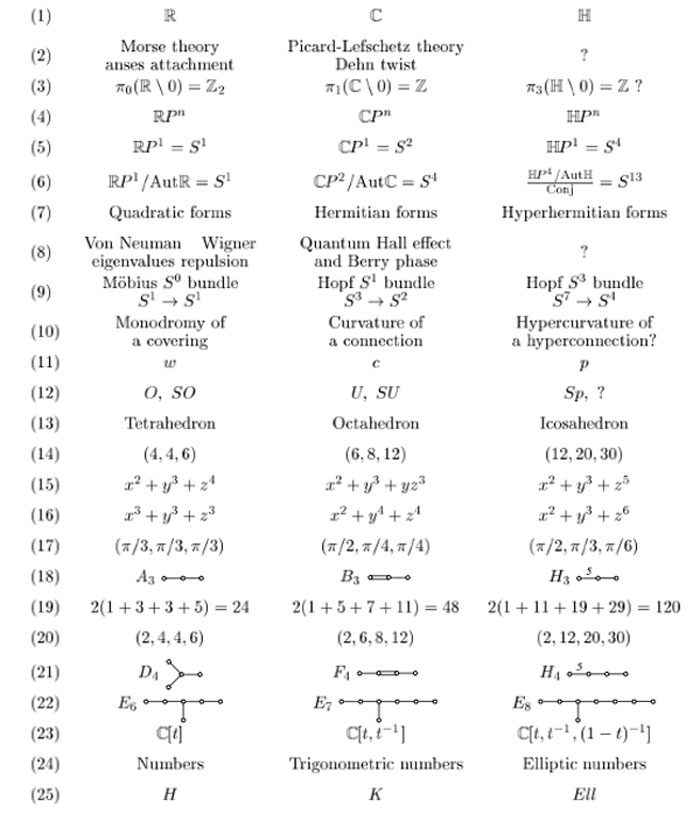I learned of this newer paper from a comment by Frederic Chapoton who maintains a nice webpage dedicated to trinities.

In his list there is one trinity on sporadic groups :where $F_{24}$ is the Fischer simple group of order $2^{21}.3^{16}.5^2.7^3.11.13.17.23.29 = 1255205709190661721292800$, which is the third largest sporadic group (the two larger ones being the Baby Monster and the Monster itself).

I don’t know what the rationale is behind this trinity. But I’d like to recall the (Baby)Monster history as a warning against the trinity-reflex. Sometimes, there is just no way to extend a would be trinity.

The story comes from Mark Ronan’s book Symmetry and the Monster on page 178.

Let’s remind ourselves how we got here. A few years earlier, Fischer has created his ‘transposition’ groups Fi22, Fi23, and Fi24. He had called them M(22), M(23), and M(24), because they were related to Mathieu’s groups M22,M23, and M24, and since he used Fi22 to create his new group of mirror symmetries, he tentatively called it $M^{22}$.
It seemed to appear as a cross-section in something even bigger, and as this larger group was clearly associated with Fi24, he labeled it $M^{24}$. Was there something in between that could be called $M^{23}$?
Fischer visited Cambridge to talk on his new work, and Conway named these three potential groups the Baby Monster, the Middle Monster, and the Super Monster. When it became clear that the Middle Monster didn’t exist, Conway settled on the names Baby Monster and Monster, and this became the standard terminology.

Marcus du Sautoy’s account in Finding Moonshine is slightly different. He tells on page 322 that the Super Monster didn’t exist. Anyone knowing the factual story?

Some mathematical trickery later revealed that the Super Monster was going to be impossible to build: there were certain features that contradicted each other. It was just a mirage, which vanished under closer scrutiny. But the other two were still looking robust. The Middle Monster was rechristened simply the Monster.

And, the inclusion diagram of the sporadic simples tells yet another story.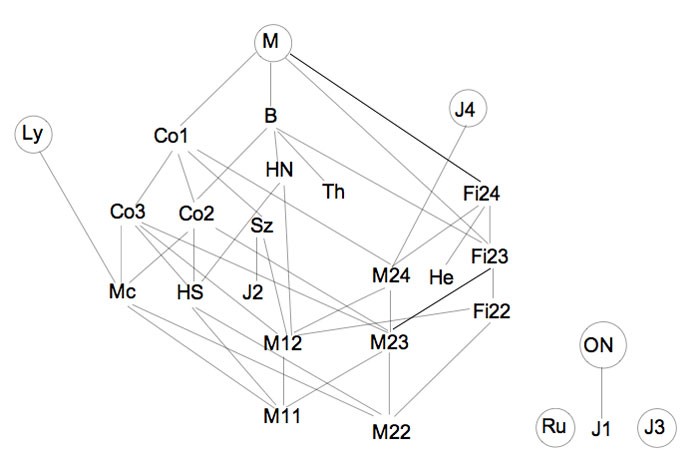Anyhow, this inclusion diagram is helpful in seeing the three generations of the Happy Family (as well as the Pariahs) of the sporadic groups, terminology invented by Robert Griess in his 100+p Inventiones paper on the construction of the Monster (which he liked to call, for obvious reasons, the Friendly Giant denoted by FG).
The happy family appears in Table 1.1. of the introduction.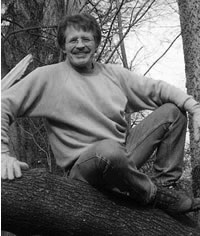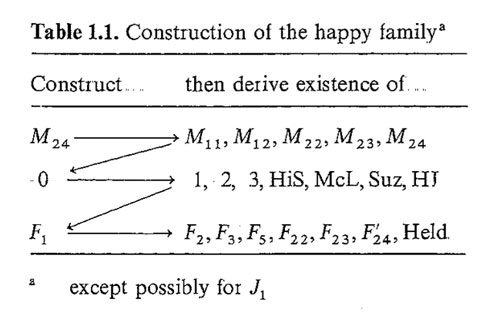It was this picture that made me propose the trinity on the left below in the previous post. I now like to add another trinity on the right, and, the connection between the two is clear.Here $Golay$ denotes the extended binary Golay code of which the Mathieu group $M_{24}$ is the automorphism group. $Leech$ is of course the 24-dimensional Leech lattice of which the automorphism group is a double cover of the Conway group $Co_1$. $Griess$ is the Griess algebra which is a nonassociative 196884-dimensional algebra of which the automorphism group is the Monster.

I am aware of a construction of the Leech lattice involving the quaternions (the icosian construction of chapter 8, section 2.2 of SPLAG). Does anyone know of a construction of the Griess algebra involving octonions???

There are only a handful of human activities where one goes to extraordinary lengths to keep a dream alive, in spite of overwhelming evidence : religion, theoretical physics, supporting the Belgian football team and … mathematics.

In recent years several people spend a lot of energy looking for properties of an elusive object : the field with one element $\mathbb{F}_1$, or in French : “F-un”. The topic must have reached a level of maturity as there was a conference dedicated entirely to it : NONCOMMUTATIVE GEOMETRY AND GEOMETRY OVER THE FIELD WITH ONE ELEMENT.

In this series I’d like to find out what the fuss is all about, why people would like it to exist and what it has to do with noncommutative geometry. However, before we start two remarks :

The field $\mathbb{F}_1$ does not exist, so don’t try to make sense of sentences such as “The ‘field with one element’ is the free algebraic monad generated by one constant (p.26), or the universal generalized ring with zero (p.33)” in the wikipedia-entry. The simplest proof is that in any (unitary) ring we have $0 \not= 1$ so any ring must contain at least two elements. A more highbrow version : the ring of integers $\mathbb{Z}$ is the initial object in the category of unitary rings, so it cannot be an algebra over anything else.

The second remark is that several people have already written blog-posts about $\mathbb{F}_1$. Here are a few I know of : David Corfield at the n-category cafe and at his old blog, Noah Snyder at the secret blogging seminar, Kea at the Arcadian functor, AC and K. Consani at Noncommutative geometry and John Baez wrote about it in his weekly finds.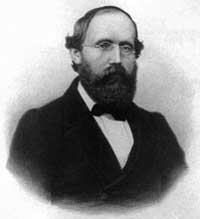The dream we like to keep alive is that we will prove the Riemann hypothesis one fine day by lifting Weil’s proof of it in the case of curves over finite fields to rings of integers.

Even if you don’t know a word about Weil’s method, if you think about it for a couple of minutes, there are two immediate formidable problems with this strategy.

For most people this would be evidence enough to discard the approach, but, we mathematicians have found extremely clever ways for going into denial.

The first problem is that if we want to think of $\mathbf{spec}(\mathbb{Z})$ (or rather its completion adding the infinite place) as a curve over some field, then $\mathbb{Z}$ must be an algebra over this field. However, no such field can exist…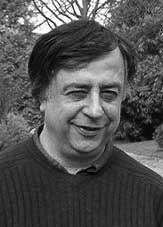No problem! If there is no such field, let us invent one, and call it $\mathbb{F}_1$. But, it is a bit hard to do geometry over an illusory field. Christophe Soule succeeded in defining varieties over $\mathbb{F}_1$ in a talk at the 1999 Arbeitstagung and in a more recent write-up of it : Les varietes sur le corps a un element.

We will come back to this in more detail later, but for now, here’s the main idea. Consider an existent field $k$ and an algebra $k \rightarrow R$ over it. Now study the properties of the functor (extension of scalars) from $k$-schemes to $R$-schemes. Even if there is no morphism $\mathbb{F}_1 \rightarrow \mathbb{Z}$, let us assume it exists and define $\mathbb{F}_1$-varieties by requiring that these guys should satisfy the properties found before for extension of scalars on schemes defined over a field by going to schemes over an algebra (in this case, $\mathbb{Z}$-schemes). Roughly speaking this defines $\mathbb{F}_1$-schemes as subsets of points of suitable $\mathbb{Z}$-schemes.

But, this is just one half of the story. He adds to such an $\mathbb{F}_1$-variety extra topological data ‘at infinity’, an idea he attributes to J.-B. Bost. This added feature is a $\mathbb{C}$-algebra $\mathcal{A}_X$, which does not necessarily have to be commutative. He only writes : “Par ignorance, nous resterons tres evasifs sur les proprietes requises sur cette $\mathbb{C}$-algebre.”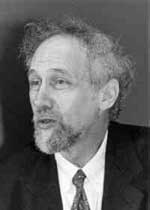The algebra $\mathcal{A}_X$ originates from trying to bypass the second major obstacle with the Weil-Riemann-strategy. On a smooth projective curve all points look similar as is clear for example by noting that the completions of all local rings are isomorphic to the formal power series $k[[x]]$ over the basefield, in particular there is no distinction between ‘finite’ points and those lying at ‘infinity’.

The completions of the local rings of points in $\mathbf{spec}(\mathbb{Z})$ on the other hand are completely different, for example, they have residue fields of different characteristics… Still, local class field theory asserts that their quotient fields have several common features. For example, their Brauer groups are all isomorphic to $\mathbb{Q}/\mathbb{Z}$. However, as $Br(\mathbb{R}) = \mathbb{Z}/2\mathbb{Z}$ and $Br(\mathbb{C}) = 0$, even then there would be a clear distinction between the finite primes and the place at infinity…

Alain Connes came up with an extremely elegant solution to bypass this problem in Noncommutative geometry and the Riemann zeta function. He proposes to replace finite dimensional central simple algebras in the definition of the Brauer group by AF (for Approximately Finite dimensional)-central simple algebras over $\mathbb{C}$. This is the origin and the importance of the Bost-Connes algebra.

We will come back to most of this in more detail later, but for the impatient, Connes has written a paper together with Caterina Consani and Matilde Marcolli Fun with $\mathbb{F}_1$ relating the Bost-Connes algebra to the field with one element.

John Conway once wrote :

There are almost as many different constructions of $M_{24}$ as there have been mathematicians interested in that most remarkable of all finite groups.

In the inguanodon post Ive added yet another construction of the Mathieu groups $M_{12}$ and $M_{24}$ starting from (half of) the Farey sequences and the associated cuboid tree diagram obtained by demanding that all edges are odd. In this way the Mathieu groups turned out to be part of a (conjecturally) infinite sequence of simple groups, starting as follows :

$L_2(7),M_{12},A_{16},M_{24},A_{28},A_{40},A_{48},A_{60},A_{68},A_{88},A_{96},A_{120},A_{132},A_{148},A_{164},A_{196},\ldots$

It is quite easy to show that none of the other sporadics will appear in this sequence via their known permutation representations. Still, several of the sporadic simple groups are generated by an element of order two and one of order three, so they are determined by a finite dimensional permutation representation of the modular group $PSL_2(\mathbb{Z})$ and hence are hiding in a special polygonal region of the Dedekind’s tessellation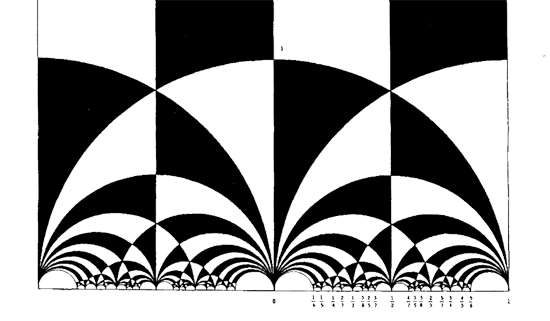Let us try to figure out where the sporadic with the next simplest permutation representation is hiding : the second Janko group $J_2$, via its 100-dimensional permutation representation. The Atlas tells us that the order two and three generators act as

e:= (1,84)(2,20)(3,48)(4,56)(5,82)(6,67)(7,55)(8,41)(9,35)(10,40)(11,78)(12, 100)(13,49)(14,37)(15,94)(16,76)(17,19)(18,44)(21,34)(22,85)(23,92)(24, 57)(25,75)(26,28)(27,64)(29,90)(30,97)(31,38)(32,68)(33,69)(36,53)(39,61) (42,73)(43,91)(45,86)(46,81)(47,89)(50,93)(51,96)(52,72)(54,74)(58,99) (59,95)(60,63)(62,83)(65,70)(66,88)(71,87)(77,98)(79,80);

v:= (1,80,22)(2,9,11)(3,53,87)(4,23,78)(5,51,18)(6,37,24)(8,27,60)(10,62,47) (12,65,31)(13,64,19)(14,61,52)(15,98,25)(16,73,32)(17,39,33)(20,97,58) (21,96,67)(26,93,99)(28,57,35)(29,71,55)(30,69,45)(34,86,82)(38,59,94) (40,43,91)(42,68,44)(46,85,89)(48,76,90)(49,92,77)(50,66,88)(54,95,56) (63,74,72)(70,81,75)(79,100,83);


But as the kfarey.sage package written by Chris Kurth calculates the Farey symbol using the L-R generators, we use GAP to find those

L = e*v^-1  and  R=e*v^-2 so

L=(1,84,22,46,70,12,79)(2,58,93,88,50,26,35)(3,90,55,7,71,53,36)(4,95,38,65,75,98,92)(5,86,69,39,14,6,96)(8,41,60,72,61,17, 64)(9,57,37,52,74,56,78)(10,91,40,47,85,80,83)(11,23,49,19,33,30,20)(13,77,15,59,54,63,27)(16,48,87,29,76,32,42)(18,68, 73,44,51,21,82)(24,28,99,97,45,34,67)(25,81,89,62,100,31,94)

R=(1,84,80,100,65,81,85)(2,97,69,17,13,92,78)(3,76,73,68,16,90,71)(4,54,72,14,24,35,11)(5,34,96,18,42,32,44)(6,21,86,30,58, 26,57)(7,29,48,53,36,87,55)(8,41,27,19,39,52,63)(9,28,93,66,50,99,20)(10,43,40,62,79,22,89)(12,83,47,46,75,15,38)(23,77, 25,70,31,59,56)(33,45,82,51,67,37,61)(49,64,60,74,95,94,98)


Defining these permutations in sage and using kfarey, this gives us the Farey-symbol of the associated permutation representation

L=SymmetricGroup(Integer(100))("(1,84,22,46,70,12,79)(2,58,93,88,50,26,35)(3,90,55,7,71,53,36)(4,95,38,65,75,98,92)(5,86,69,39,14,6,96)(8,41,60,72,61,17, 64)(9,57,37,52,74,56,78)(10,91,40,47,85,80,83)(11,23,49,19,33,30,20)(13,77,15,59,54,63,27)(16,48,87,29,76,32,42)(18,68, 73,44,51,21,82)(24,28,99,97,45,34,67)(25,81,89,62,100,31,94)")

R=SymmetricGroup(Integer(100))("(1,84,80,100,65,81,85)(2,97,69,17,13,92,78)(3,76,73,68,16,90,71)(4,54,72,14,24,35,11)(5,34,96,18,42,32,44)(6,21,86,30,58, 26,57)(7,29,48,53,36,87,55)(8,41,27,19,39,52,63)(9,28,93,66,50,99,20)(10,43,40,62,79,22,89)(12,83,47,46,75,15,38)(23,77, 25,70,31,59,56)(33,45,82,51,67,37,61)(49,64,60,74,95,94,98)")

sage: FareySymbol("Perm",[L,R])

[[0, 1, 4, 3, 2, 5, 18, 13, 21, 71, 121, 413, 292, 463, 171, 50, 29, 8, 27, 46, 65, 19, 30, 11, 3, 10, 37, 64, 27, 17, 7, 4, 5], [1, 1, 3, 2, 1, 2, 7, 5, 8, 27, 46, 157, 111, 176, 65, 19, 11, 3, 10, 17, 24, 7, 11, 4, 1, 3, 11, 19, 8, 5, 2, 1, 1], [-3, 1, 4, 4, 2, 3, 6, -3, 7, 13, 14, 15, -3, -3, 15, 14, 11, 8, 8, 10, 12, 12, 10, 9, 5, 5, 9, 11, 13, 7, 6, 3, 2, 1]]


Here, the first string gives the numerators of the cusps, the second the denominators and the third gives the pairing information (where [tex[-2 $denotes an even edge and$-3 $an odd edge. Fortunately, kfarey also allows us to draw the special polygonal region determined by a Farey-symbol. So, here it is (without the pairing data) :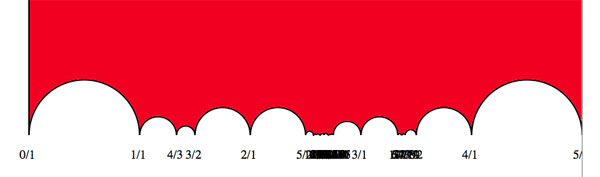the hiding place of$J_2 $… It would be nice to have (a) other Farey-symbols associated to the second Janko group, hopefully showing a pattern that one can extend into an infinite family as in the inguanodon series and (b) to determine Farey-symbols of more sporadic groups. Towards the end of the Bost-Connes for ringtheorists post I freaked-out because I realized that the commutation morphisms with the$X_n^* $were given by non-unital algebra maps. I failed to notice the obvious, that algebras such as$\mathbb{Q}[\mathbb{Q}/\mathbb{Z}] $have plenty of idempotents and that this mysterious ‘non-unital’ morphism was nothing else but multiplication with an idempotent… Here a sketch of a ringtheoretic framework in which the Bost-Connes Hecke algebra$\mathcal{H} $is a motivating example (the details should be worked out by an eager 20-something). Start with a suitable semi-group$S $, by which I mean that one must be able to invert the elements of$S $and obtain a group$G $of which all elements have a canonical form$g=s_1s_2^{-1} $. Probably semi-groupies have a name for these things, so if you know please drop a comment. The next ingredient is a suitable ring$R $. Here, suitable means that we have a semi-group morphism$\phi~:~S \rightarrow End(R) $where$End(R) $is the semi-group of all ring-endomorphisms of$R $satisfying the following two (usually strong) conditions : 1. Every$\phi(s) $has a right-inverse, meaning that there is an ring-endomorphism$\psi(s) $such that$\phi(s) \circ \psi(s) = id_R $(this implies that all$\phi(s) $are in fact epi-morphisms (surjective)), and 2. The composition$\psi(s) \circ \phi(s) $usually is NOT the identity morphism$id_R $(because it is zero on the kernel of the epimorphism$\phi(s) $) but we require that there is an idempotent$E_s \in R $(that is,$E_s^2 = E_s $) such that$\psi(s) \circ \phi(s) = id_R E_s $The point of the first condition is that the$S $-semi-group graded ring$A = \oplus_{s \in S} X_s R $is crystalline graded (crystalline group graded rings were introduced by Fred Van Oystaeyen and Erna Nauwelaarts) meaning that for every$s \in S $we have in the ring$A $the equality$X_s R = R X_s $where this is a free right$R $-module of rank one. One verifies that this is equivalent to the existence of an epimorphism$\phi(s) $such that for all$r \in R $we have$r X_s = X_s \phi(s)(r) $. The point of the second condition is that this semi-graded ring$A$can be naturally embedded in a$G $-graded ring$B = \oplus_{g=s_1s_2^{-1} \in G} X_{s_1} R X_{s_2}^* $which is bi-crystalline graded meaning that for all$r \in R $we have that$r X_s^*= X_s^* \psi(s)(r) E_s $. It is clear from the construction that under the given conditions (and probably some minor extra ones making everything stand) the group graded ring$B $is determined fully by the semi-group graded ring$A $. what does this general ringtheoretic mumbo-jumbo have to do with the BC- (or Bost-Connes) algebra$\mathcal{H} $? In this particular case, the semi-group$S $is the multiplicative semi-group of positive integers$\mathbb{N}^+_{\times} $and the corresponding group$G $is the multiplicative group$\mathbb{Q}^+_{\times} $of all positive rational numbers. The ring$R $is the rational group-ring$\mathbb{Q}[\mathbb{Q}/\mathbb{Z}] $of the torsion-group$\mathbb{Q}/\mathbb{Z} $. Recall that the elements of$\mathbb{Q}/\mathbb{Z} $are the rational numbers$0 \leq \lambda < 1 $and the group-law is ordinary addition and forgetting the integral part (so merely focussing on the ‘after the comma’ part). The group-ring is then$\mathbb{Q}[\mathbb{Q}/\mathbb{Z}] = \oplus_{0 \leq \lambda < 1} \mathbb{Q} Y_{\lambda} $with multiplication linearly induced by the multiplication on the base-elements$Y_{\lambda}.Y_{\mu} = Y_{\lambda+\mu} $. The epimorphism determined by the semi-group map$\phi~:~\mathbb{N}^+_{\times} \rightarrow End(\mathbb{Q}[\mathbb{Q}/\mathbb{Z}]) $are given by the algebra maps defined by linearly extending the map on the base elements$\phi(n)(Y_{\lambda}) = Y_{n \lambda} $(observe that this is indeed an epimorphism as every base element$Y_{\lambda} = \phi(n)(Y_{\frac{\lambda}{n}}) $. The right-inverses$\psi(n) $are the ring morphisms defined by linearly extending the map on the base elements$\psi(n)(Y_{\lambda}) = \frac{1}{n}(Y_{\frac{\lambda}{n}} + Y_{\frac{\lambda+1}{n}} + \ldots + Y_{\frac{\lambda+n-1}{n}}) $(check that these are indeed ring maps, that is that$\psi(n)(Y_{\lambda}).\psi(n)(Y_{\mu}) = \psi(n)(Y_{\lambda+\mu}) $. These are indeed right-inverses satisfying the idempotent condition for clearly$\phi(n) \circ \psi(n) (Y_{\lambda}) = \frac{1}{n}(Y_{\lambda}+\ldots+Y_{\lambda})=Y_{\lambda} $and$\begin{eqnarray} \psi(n) \circ \phi(n) (Y_{\lambda}) =& \psi(n)(Y_{n \lambda}) = \frac{1}{n}(Y_{\lambda} + Y_{\lambda+\frac{1}{n}} + \ldots + Y_{\lambda+\frac{n-1}{n}}) \\ =& Y_{\lambda}.(\frac{1}{n}(Y_0 + Y_{\frac{1}{n}} + \ldots + Y_{\frac{n-1}{n}})) = Y_{\lambda} E_n \end{eqnarray} $and one verifies that$E_n = \frac{1}{n}(Y_0 + Y_{\frac{1}{n}} + \ldots + Y_{\frac{n-1}{n}}) $is indeed an idempotent in$\mathbb{Q}[\mathbb{Q}/\mathbb{Z}] $. In the previous posts in this series we have already seen that with these definitions we have indeed that the BC-algebra is the bi-crystalline graded ring$B = \mathcal{H} = \oplus_{\frac{m}{n} \in \mathbb{Q}^+_{\times}} X_m \mathbb{Q}[\mathbb{Q}/\mathbb{Z}] X_n^* $and hence is naturally constructed from the skew semi-group graded algebra$A = \oplus_{m \in \mathbb{N}^+_{\times}} X_m \mathbb{Q}[\mathbb{Q}/\mathbb{Z}] $. This (probably) explains why the BC-algebra$\mathcal{H} $is itself usually called and denoted in$C^* $-algebra papers the skew semigroup-algebra$\mathbb{Q}[\mathbb{Q}/\mathbb{Z}] \bowtie \mathbb{N}^+_{\times} $as this subalgebra (our crystalline semi-group graded algebra$A $) determines the Hecke algebra completely. Finally, the bi-crystalline idempotents-condition works well in the settings of von Neumann regular algebras (such as all limits of finite dimensional semi-simples, for example$\mathbb{Q}[\mathbb{Q}/\mathbb{Z}] $) because such algebras excel at idempotents galore A classic Andre Weil-tale is his narrow escape from being shot as a Russian spy The war was a disaster for Weil who was a conscientious objector and so wished to avoid military service. He fled to Finland, to visit Rolf Nevanlinna, as soon as war was declared. This was an attempt to avoid being forced into the army, but it was not a simple matter to escape from the war in Europe at this time. Weil was arrested in Finland and when letters in Russian were found in his room (they were actually from Pontryagin describing mathematical research) things looked pretty black. One day Nevanlinna was told that they were about to execute Weil as a spy, and he was able to persuade the authorities to deport Weil instead.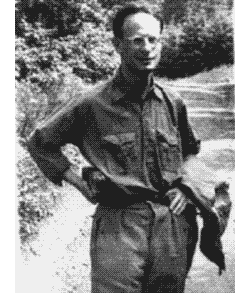However, Weil’s wikipedia entry calls this a story too good to be true, and continues In 1992, the Finnish mathematician Osmo Pekonen went to the archives to check the facts. Based on the documents, he established that Weil was not really going to be shot, even if he was under arrest, and that Nevanlinna probably didn’t do – and didn’t need to do – anything to save him. Pekonen published a paper on this with an afterword by Andre Weil himself. Nevanlinna’s motivation for concocting such a story of himself as the rescuer of a famous Jewish mathematician probably was the fact that he had been a Nazi sympathizer during the war. The story also appears in Nevanlinna’s autobiography, published in Finnish, but the dates don’t match with real events at all. It is true, however, that Nevanlinna housed Weil in the summer of 1939 at his summer residence Korkee at Lohja in Finland – and offered Hitler’s Mein Kampf as bedside reading. This old spy-story gets a recent twist now that it turns out that Weil’s descent theory of tori has applications to cryptography. So far, I haven’t really defined what tori are, so let us start with some basics. The simplest (and archetypical) example of an algebraic torus is the multiplicative group(scheme)$\mathbb{G}_m $over a finite field$\mathbb{F}_q $which is the affine variety$\mathbb{V}(xy-1) \subset \mathbb{A}^2_{\mathbb{F}_q} $. that is, the$\mathbb{F}_q $points of$\mathbb{G}_m $are precisely the couples${ (x,\frac{1}{x})~:~x \in \mathbb{F}_q^* } $and so are in one-to-one correspondence with the non-zero elements of$\mathbb{F}_q $. The coordinate ring of this variety is the ring of Laurant polynomials$\mathbb{F}_q[x,x^{-1}] $and the fact that multiplication induces a group-structure on the points of the variety can be rephrased by saying that this coordinate ring is a Hopf algebra which is just the Hopf structure on the group-algebra$\mathbb{F}_q[\mathbb{Z}] = \mathbb{F}_q[x,x^{-1}] $. This is the first indication of a connection between tori defined over$\mathbb{F}_q $and lattices (that is free$\mathbb{Z} $-modules with an action of the Galois group$Gal(\overline{F}_q/F_q) $. In this correspondence, the multiplicative group scheme$\mathbb{G}_m $corresponds to$\mathbb{Z} $with the trivial action. Now take a field extension$\mathbb{F}_q \subset \mathbb{F}_{q^n} $, is there an affine variety, defined over$\mathbb{F}_q $whose$\mathbb{F}_q $-points are precisely the invertible elements$\mathbb{F}_{q^n}^* $? Sure! Just take the multiplicative group over$\mathbb{F}_{q^n} $and write the elements x and y as$x = x_1 + x_2 a_2 + \ldots + x_n a_n $(and a similar expression for y with${ 1,a_2,\ldots,a_n }$being a basis of$\mathbb{F}_{q^n}/\mathbb{F}_q $and write the defning equation$xy-1 $out, also with respect to this basis and this will then give you the equations of the desired variety, which is usually denoted by$R^1_{\mathbb{F}_{q^n}/\mathbb{F}_q} \mathbb{G}_m $and called the Weil restriction of scalars torus. A concrete example? Take$\mathbb{F}_9 = \mathbb{F}_3(\sqrt{-1}) $and write$x=x_1+x_2 \sqrt{-1} $and$y=y_1+y_2 \sqrt{-1} $, then the defining equation$xy-1 $becomes$~(x_1y_1-x_2y_2) + (x_1y_2-x_2y_1) \sqrt{-1} = 1 $whence$R^1_{\mathbb{F}_9/\mathbb{F}_3} = \mathbb{V}(x_1y_1-x_2y_2-1,x_1y_2-x_2y_1) \subset \mathbb{A}^4_{\mathbb{F}_3} $, the intersection of two quadratic hypersurfaces in 4-dimensional space. Why do we call$R^1 \mathbb{G}_m $a _torus_? Well, as with any variety defined over$\mathbb{F}_q $we can also look at its points over a field-extension, for example over the algebraic closure$\overline{\mathbb{F}}_q $and then it is easy to see that$R^1_{\mathbb{F}_{q^n}/\mathbb{F}_q} \mathbb{G}_m (\overline{\mathbb{F}}_q) = \overline{\mathbb{F}}_q^* \times \ldots \times \overline{\mathbb{F}}_q^* $(n copies) and such algebraic groups are called tori. (To understand terminology, the compact group corresponding to$\mathbb{C}^* \times \mathbb{C}^* $is$U_1 \times U_1 = S^1 \times S^1 $, so a torus). In fact, it is already the case that the$\mathbb{F}_{q^n} $points of the restriction of scalar torus are$\mathbb{F}_{q^n}^* \times \ldots \times \mathbb{F}_{q^n}^* $and therefore we call this field a splitting field of the torus. This is the general definition of an algebraic torus : a torus T over$\mathbb{F}_q $is an affine group scheme over$\mathbb{F}_q $such that, if we extend scalars to the algebraic closure (and then it already holds for a finite extension) we get an isomorphism of affine group schemes$T \times_{\mathbb{F}_q} \overline{\mathbb{F}}_q = \overline{\mathbb{F}}_q^* \times \ldots \times \overline{\mathbb{F}}_q^* = (\overline{\mathbb{F}}_q^*)^{n} $in which case we call T a torus of dimension n. Clearly, the Galois group$Gal(\overline{\mathbb{F}}_q^*/\mathbb{F}_q) $acts on the left hand side in such a way that we recover$T $as the orbit space for this action. Hence, anther way to phrase this is to say that an algebraic torus is the Weil descent of an action of the Galois group on the algebraic group$\overline{\mathbb{F}}_q^* \times \ldots \times \overline{\mathbb{F}}_q^* $. Of course we can also rephrase this is more algebraic terms by looking at the coordinate rings. The coordinate ring of the algebraic group$~(\overline{\mathbb{F}}_q^*)^n $is the group-algebra of the rank n lattice$\mathbb{Z}^n = \mathbb{Z} \oplus \ldots \oplus \mathbb{Z} $(the free Abelian group of rank n), that is,$\overline{\mathbb{F}}_q [ \mathbb{Z}^n ] $. Now the Galois group acts both on the field$\overline{\mathbb{F}}_q $as on the lattice$\mathbb{Z}^n $coming from the action of the Galois group on the extended torus$T \times_{\mathbb{F}_q} \overline{\mathbb{F}}_q $. In fact, it is best to denote this specific action on$\mathbb{Z}^n $by$T^* $and call$T^* $the character group of$T $. Now, we recover the coordinate ring of the$\mathbb{F}_q $-torus$T $as the ring of invariants$\mathbb{F}_q[T] = \overline{\mathbb{F}}_q [T^*]^{Gal(\overline{\mathbb{F}}_q/\mathbb{F}_q)} $Hence, the restriction of scalars torus$R^1_{\mathbb{F}_{q^n}/\mathbb{F}_q} \mathbb{G}_m $is an n-dimensional torus over$\mathbb{F}_q $and its corresponding character group is the free Abelian group of rank n which can be written as$\mathbb{Z}[x]/(x^n-1) = \mathbb{Z}1 \oplus \mathbb{Z}x \oplus \ldots \oplus \mathbb{Z}x^{n-1} $and where the action of the cyclic Galois group$Gal(\mathbb{F}_{q^n}/\mathbb{F}_q) = C_n = \langle \sigma \rangle $s such that the generator$\sigma $as as multiplication by$x $. That is, in this case the character group is a permutation lattice meaning that the$\mathbb{Z} $-module has a basis which is permuted under the action of the Galois group. Next time we will encounter more difficult tori sich as the crypto-torus$T_n \$.